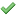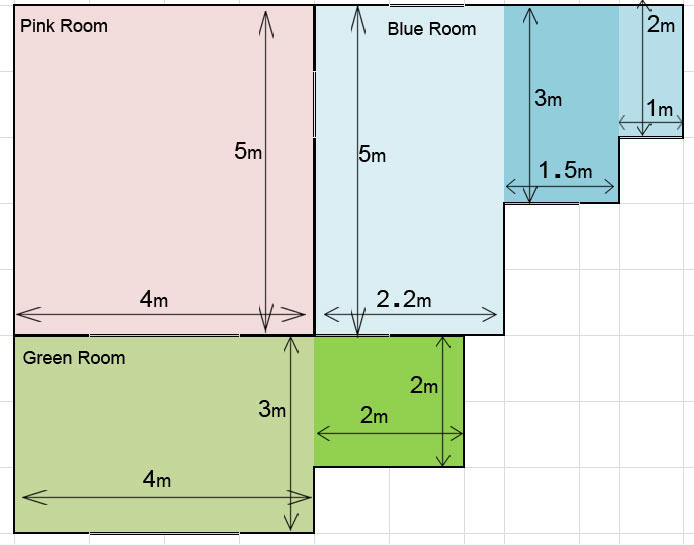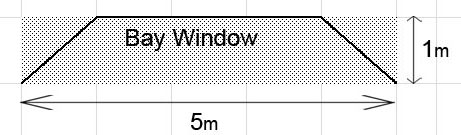WFD12
Showroom open Monday-Friday 9am-5pm, Saturday 10am-4pm## How to measure your floor

OK so you have found the floor you want. So how much do you need to order and how much will it cost. Best to get hold of a tape measure, paper and pen Also maybe a calculator. Draw the shape of the roomsHOW TO CALCULATE YOUR FLOOR AREA
PINK ROOM    If you have a rectangular or square room measure length x width and multiply the two as shown here 5m x 4m =20m2
GREEN ROOM    If you have an L shaped room just divide it into two areas 4 x 3 = 12m2  and 2 x 2 = 4m2. Then just add the two together 12 + 4 = 16m2
BLUE ROOM    If you have an odd shaped room divide it into multiple areas ie: 5 x 2.2 = 11m2 3 x 1.5 = 4.5m2 and 2 x 1 = 2m2. Then add the different m2 areas together  11 + 4.5 + 2 = 17.5m2

Don’t forget you will probably need to allow a little bit more for wastage!. Often the recommended wastage is 5%. Simply add the three rooms together20m2 + 16m2 + 17.5m2 = 53.5m2 plus 5% = 56.17m2BAY WINDOW  If you have a small angled area such as a Bay window, just multiply the maximum width by the depth. 5 x 1 = 5m2

Top

Hamiltons Doors and Floors is a trading name of Hamiltons the Home Improvement Company Ltd. (Hamiltons)
a company registered in England No. 3041656, VAT No. GB637074141

### Mailing List